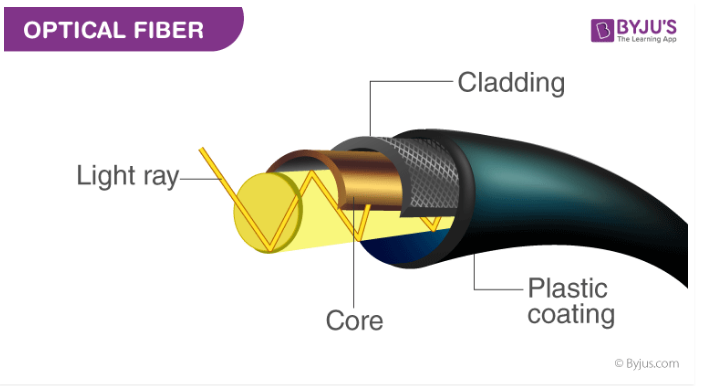Win up to 100% scholarship on Aakash BYJU'S JEE/NEET courses with ABNAT Win up to 100% scholarship on Aakash BYJU'S JEE/NEET courses with ABNAT

# Fibre Optics MCQs for NEET

Optical fibre is the technology of data transmission using light pulses travelling through the long fibre which is mostly made of glass or plastic. Optical fibres are not affected by electromagnetic interference. The optical fibre works on the principle of total internal reflection of light. The numerical aperture of optical fibre can be defined as a measure of the ability of light collection by the fibre. Single-mode fibre is used for long-distance transmission while multimode fibre is used for shorter distances.Q1: Data signal with minimum error is generated by which among the following

1. Signal processing circuits
2. Photodiode
3. Linear circuitry
4. None of the above

Q2: Which among the following is described by the concept of numerical aperture in an optical fibre?

1. Light collection
2. Light scattering
3. Light dispersion
4. Light polarisation

Note: The numerical aperture of optical fibre can be defined as a measure of the ability of light collection by the fibre.

Q3: An optical fibre consists of a core μ1 surrounded by a cladding of μ＜ μ1. A beam of light enters from the air at an angle of α with the axis of the fibre. The highest α for which ray can be travelled through fibre is

1. $$\begin{array}{l}cos^{-1}\sqrt{{\mu_{2}}^{2} -{\mu_{1}}^{2}}\end{array}$$
2. $$\begin{array}{l}sin^{-1}\sqrt{{\mu_{1}}^{2} -{\mu_{2}}^{2}}\end{array}$$
3. $$\begin{array}{l}tan^{-1}\sqrt{{\mu_{1}}^{2} -{\mu_{2}}^{2}}\end{array}$$
4. $$\begin{array}{l}sec^{-1}\sqrt{{\mu_{1}}^{2} -{\mu_{2}}^{2}}\end{array}$$

$$\begin{array}{l}sin^{-1}\sqrt{{\mu_{1}}^{2} -{\mu_{2}}^{2}}\end{array}$$

Q4: In an optical fibre communication system, which among the following is not a typical transmitter function?

1. Coding for error protection
2. Decoding of input data
3. Electrical to optical conversion
4. Recoding to match output standard

Answer: (d) Recoding to match output standard

Q5: In a single-mode fibre, how does the fraction of energy travelling through bound mode appear in the cladding?

1. As a crescent wave
2. As a gibbous wave
3. As an evanescent wave
4. All the above

Answer: (c) As an evanescent wave

Q6: Which among the following fibre optic cables have a core of size 480 μm to 980 μm and made up of polymethylmethacrylate?

1. Glass fibre optic cable
2. Plastic fibre optic cable
3. Plastic clad silica fibre optic cable
4. All of the above

Answer: (b) Plastic fibre optic cable

Q7: A ray of light will undergo total internal reflection if it

1. Goes from rarer medium to denser medium
2. Incident at an angle less than the critical angle
3. Strikes the interface normally
4. Incident at an angle greater than the critical angle

Answer: (d) Incident at an angle greater than the critical angle

Q8: Which of the following is not due to total internal reflection of light?

1. Brilliance of diamond
2. Mirage formation
3. Optical fibre working
4. Rainbow formation

Q9: The fibres not used nowadays for optical fibre communication system are

1. Single-mode fibre
2. Multimode fibre
3. Coaxial cable

Q10: In single-mode fibres, the cladding diameter must be at least

1. Five times the core diameter
2. Thrice the core diameter
3. Ten times the core diameter
4. Twice the core diameter

Answer: (c) Ten times the core diameter

Also check: